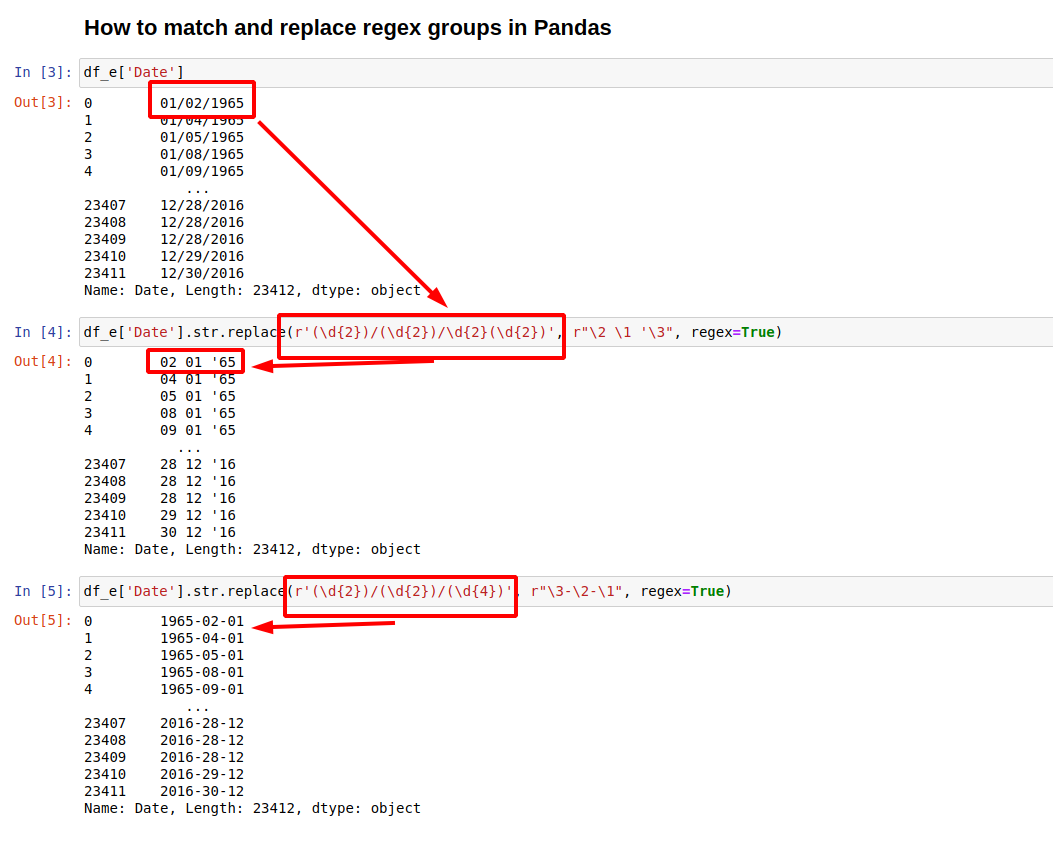In this short tutorial, we'll look at how to match and replace regex groups in Pandas.

Here you can find the short answer:

``````df_e['Date'].str.replace(r'(\d{2})/(\d{2})/(\d{4})', r"\3-\2-\1", regex=True)
``````

## How to match and replace regex groups in Pandas

Let's check first for the regex pattern `r'(\d{2})/(\d{2})/(\d{4})'`. It has 3 capturing groups::

• 1st capturing group `(\d{2})`
• `\d` - matches a digit (equivalent to [0-9])
• {2} matches the previous token exactly 2 times
• 2nd capturing group `(\d{2})`
• `\d` - matches a digit (equivalent to [0-9])
• {2} matches the previous token exactly 2 times
• 4nd capturing group `(\d{4})`
• `\d` - matches a digit (equivalent to [0-9])
• {4} matches the previous token exactly 4 times

Between the capturing groups there are separators - `/` which will be matched but not captured.

Now in the replacement part - `r"\3-\2-\1"` - the groups are presented by numbers - starting from 1:

• 1st group is `\1`
• 2nd group is `\2`
• 3rd group is `\3`

So if the initial value in Pandas column is:

``````0        01/02/1965
1        01/04/1965
2        01/05/1965
3        01/08/1965
4        01/09/1965
``````

After the replacement we will end with:

``````0        1965-02-01
1        1965-04-01
2        1965-05-01
3        1965-08-01
4        1965-09-01
``````

Another example is:

``````df_e['Date'].str.replace(r'(\d{2})/(\d{2})/\d{2}(\d{2})', r"\2 \1 '\3", regex=True)
``````

result will be:

``````0        02 01 '65
1        04 01 '65
2        05 01 '65
3        08 01 '65
4        09 01 '65
``````## How to match and replace regex groups - string patterns

Let's have string data which has a different number of words. Suppose we like to extract only the last word from all rows:

``````0                Noida
1                Noida
2       Work From Home
3       Work From Home
4       Work From Home
...
``````

We can use regex groups to match the last word by:

``````df['location'].str.replace(r'(.*) (.*) (.*)', r"\3", regex=True)
``````

result:

``````0          Noida
1          Noida
2           Home
3           Home
4           Home
``````

If you need to work with special characters and complex expressions - you may need to test a lot - in order to avoid problems.

Let's have data like:

``````0                                        Software Testing
1       Java, SQL, Unix, Oracle, MS SQL Server, Hibern...
2       English Proficiency (Spoken), English Proficie...
3                        HTML, CSS, Flask, Python, Django
4                   HTML, CSS, JavaScript, ReactJS, Redux
``````

For example if you expect:

``````df['skills'].str.replace(r'(.*)(\(.*\))(.*)', r"\1\3", regex=True)
``````

The regex above to remove all words surrounded by `(` and `)` - this will not happen:

``````0                                        Software Testing
1       Java, SQL, Unix, Oracle, MS SQL Server, Hibern...
2       English Proficiency (Spoken), English Proficie...
3                        HTML, CSS, Flask, Python, Django
4                   HTML, CSS, JavaScript, ReactJS, Redux
``````

because the regex are greedy by default and will try to match as much as possible. You can check what is captured as group 2:

``````df['skills'].str.replace(r'(.*)(\(.*\))(.*)', r"\2", regex=True)
``````

So as you can if there are multiple matches - the regex will try to match everything until the end - or matching the last one possible:

``````0                            Software Testing
1                                      (Java)
2                                   (Written)
3            HTML, CSS, Flask, Python, Django
4       HTML, CSS, JavaScript, ReactJS, Redux
``````

For non greedy matching in Pandas regex groups you can add `?`. So:

• `(.*)` - greedy match
• `(.*)` - non greedy match
``````df['skills'].str.replace(r'(.*?)(\(.*?\))(.*)', r"\2", regex=True)
``````

result:

``````0                            Software Testing
1                                      (Java)
2                                    (Spoken)
3            HTML, CSS, Flask, Python, Django
4       HTML, CSS, JavaScript, ReactJS, Redux
``````

Note that the third line changed from `(Written)` to `(Spoken)`.

## How to match and replace regex groups - numeric patterns

Suppose we have ranged amounts like:

``````0            8000 /month
1           10000 /month
2       1000-2000 /month
3       1000-2000 /month
4       1000-2000 /month
``````

And we need to get only the upper amount from this range and leave the rest as it is. This can be achieved by:

``````df['stipend'].str.replace(r'(\d+)-(\d+)(.*)', r"\2\3", regex=True)
``````

result:

``````0        8000 /month
1       10000 /month
2        2000 /month
3        2000 /month
4        2000 /month
``````